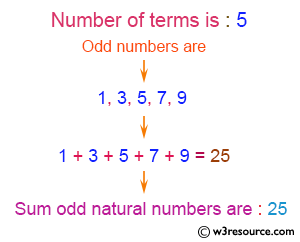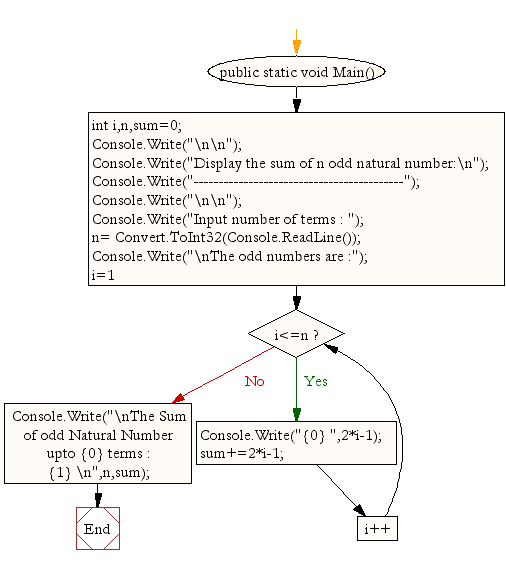﻿ C# - Display the sum of n number of odd natural number# C# Sharp Exercises: Display the sum of n number of odd natural number

## C# Sharp For Loop: Exercise-8 with Solution

Write a program in C# Sharp to display the n terms of odd natural number and their sum like:
1 3 5 7 ... n

Pictorial Presentation:Sample Solution:

C# Sharp Code:

``````using System;
public class Exercise8
{
public static void Main()
{
int i,n,sum=0;
Console.Write("\n\n");
Console.Write("Display the sum of n odd natural number:\n");
Console.Write("------------------------------------------");
Console.Write("\n\n");

Console.Write("Input number of terms : ");
Console.Write("\nThe odd numbers are :");
for(i=1;i<=n;i++)
{
Console.Write("{0} ",2*i-1);
sum+=2*i-1;
}
Console.Write("\nThe Sum of odd Natural Number upto {0} terms : {1} \n",n,sum);
}
}
```
```

Sample Output:

```Display the sum of n odd natural number:
------------------------------------------

Input number of terms : 5

The odd numbers are :1 3 5 7 9
The Sum of odd Natural Number upto 5 terms : 25
```

Flowchart:C# Sharp Code Editor:

Contribute your code and comments through Disqus.

What is the difficulty level of this exercise?

Test your Programming skills with w3resource's quiz.

﻿